Maths
Numeral systems
Author
Marion Montocchio, Lycée Charles Baudelaire
Target Group
15-17 years old students
Brief Description
Numeral systems

Share

NUMERAL SYSTEMS

A decimal system (also called base ten or occasionally denary) has ten as its base. It is the numerical base most widely used by civilizations.

 For example the decimal 5678 represents         5 × 103    +   6 × 102   +   7 × 101    +   8 × 100                                                                                 = 5 x 1000 +   6 x 100   +   7 x 10      +   8

It generally uses a new symbol for each new power of 10. Then  1  10  100  1000 have a different symbol.

 For instance, Ancient Egyptians used these numerals,  written in hyeroglyphic script.They used two ones to represent two, three ones for three, and so on, up to nine.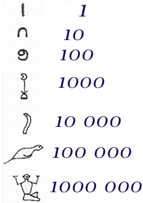Write 5678 using Ancient Egyptians numerals.         Problems : - It may take a long time to write a number.                       - You need a new symbol for any new power of 10.

 As another example, Roman numerals have symbols for the decimal powers (1, 10, 100, 1000) andsecondary symbols for half these values (5, 50, 500).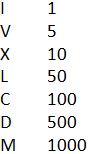Write 5678 using Ancient Egyptians numerals.  Problems : - It may take a long time to write a number.                      - You need a new symbol for any new power of 10.

Why 10 ? Probably because ten is the number which is the count of fingers and thumbs on both hands (or toes on the feet).

But some cultures do, or did, use other bases of numbers.

A positional system only needs a few symbols to represent any number, no matter how large it is.

A positional number system is one where the numbers are arranged in columns and then the value of one symbol depends on its place.

 Babylonians used stylus made of reed to write cuneiform symbols onto a wet clay tablet, which later they baked if they wished to preserve what was written on it. The number of elementary symbols is very small - there are only few lines put together in different ways - but their combinations give extremely large number of possibilities. Moreover, they tended to arrange the symbols into neat piles.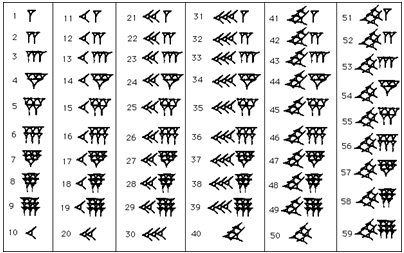The Babylonian sexagesimal  positional system (base-60) places numbers with the same convention as ours, so the right most position is for the units up to 59, the position one to the left is for 60 × n where 1 ? n ? 59, etc. If we adopt a notation where we separate the numerals by commas so, for example, 1,57,46,40 represents the sexagesimal number 1 × 603 + 57 × 602 + 46 × 60 + 40  which, in decimal notation is 424000.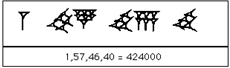There is a problem : the symbol for sixty seems to be exactly the same as that for one. Sixty one is sixty and one, which therefore looks like one and one, and so on.  Surely this is very confusing!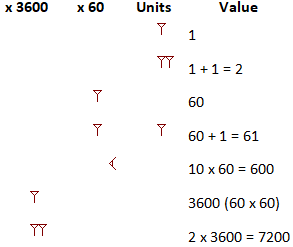You can now see why they piled the units up into neat piles! They needed to distinguish one plus one or two, from one times sixty plus one meaning sixty one. Both these have two symbols for one. But the representation of two has the two ones touching, while the representation for sixty one has a gap between them. A careless clerk might make mistakes that way, but if you were careful, it should be all right.

Believe it or not, this didn't worry them. After all, if you were counting things, you would tend to know if you were counting individual things or counting in lots of sixty (or even 3,600!) So the Babylonians didn't bother with a zero at the end of the number.

However, it is more serious with gaps in the middle of the number. The number 3601 is not too different from 3660, and they are both written as two ones. You could say that there should be a bigger gap for 3601, since the gap represents nothing in the sixty column, but how easy to make a mistake! So the Babylonians DID have a zero, which they used only in only in the middle of numbers.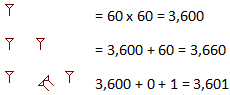The great advantage of the positional system is that you need only a limited number of symbols (the Babylonians only had two, plus their symbol for zero) and you can represent any whole number, however big.  You can also do arithmetic far easier. The Babylonians had a sophisticated number system.

 Write 5678 using Babylonians numerals.

Base 60 in modern times

You many wonder why they seemed to like the number sixty so much. Sixty is a very good number for a base. The number of divisors was maximised.  10 is divisible by  2 5

60 is divisible by 2 3 4 5 6 10 12 15 20 30

In fact, the Babylonians have given their base 60 to us. There are 60 minutes in an hour, and 60 seconds in a minute. There are also 360 degrees in a circle (6 x 60), and a single degree can be broken down still further. There are 60 minutes in a degree, and 60 seconds in one of these minutes. (There is no connection between angle minutes & seconds and time minutes & seconds.)

Babylonians were the first people to record the calendar, with the day divided into 24 hours (as we do), each hour into 60 minutes and each minute into 60 seconds.

We use a positional decimal systems : the Hindu-Arabic numeral system.

The symbols for the digits in common use around the globe today are called Arabic numerals by Europeans and Indian numerals by Arabs, the two groups' terms both referring to the culture from which they learned the system.

The Hindus in India invented this system from about the 4th century BC onwards.

This number system spread to the Middle East in about the 9th century AD, where it was used by Arab mathematicians and astronomers. (Muslim scientists used the Babylonian number system, and merchants used the Abjad numerals, a system based on letters, rather like Greek numbers.)

Arabic numbers then spread to Europe. Before this, Europeans were using Roman numbers, with abacuses for calculation. Fibonacci wrote a book about Arabic numbers in the thirteenth century AD. At first, these numbers were very unpopular in Europe, since people were used to using abacuses where you could watch the calculation taking place. But they soon realised how much easier it was to do calculations with Arabic numbers. Now Arabic numbers are generally used throughout the world for calculation.

And today computers use a base-2 system also called binary system.

With this system, calculations are very easy to compute.

Binary addition works exactly like decimal. You line up the numbers, and add up each column, starting from the right. However, it is much easier, as the only possible additions are 0+0=0, 0+1=1, 1+0=1, 1+1=10. You do need to carry, but that doesn't complicate things much.

Binary multiplication doesn't involve any multiplication tables at all. All you need are 0x0=0, 1x0=0, 0x1=0, 1x1=1. For long multiplication, you do need to add. It works like decimal multiplication. You separate one number into its powers of two (if it was a decimal number, this would be separating the number into its hundreds, tens and units, etc., so 3426 = 3000 + 400 + 20 + 6). Then you multiply the other number by each digit. You need the add the right number of '0's on the end, of course.

Numeral systems

Exercise 1 : base a to base b

Write the value 11110(base 2) in base 10 :

Write the number 25(base 10) in base 2 :

Write the number 97(base 10) in base 5 :

Write the number 33(base 4) in base 10 :

Write the number 20BF3(base 16) in base 10 :

Exercise 2 : base 2

The following numbers are given in base 2.

Compute the sum   1011 + 101

Compute the sum   1010101010111001 + 1111011011011110

Compute the subtraction   11001101 - 1001011

Compute the product  110001 x 11

CHANGE OF BASE

How to write 424 000 in base 60 ? Method 1

 ?My first step is to find the largest power of 60 that is less than 424 000. So let’s go through the powers of 60:  60, 60²=3 600, 603=216 000, 604=12 960 000 … Ok, 12 960 000  is larger than 424 000 so we take one step back and get 603. 603 is the largest power of 2 that is still smaller than 424 000. ?How many “whole” (not partial or fractional) times can 603 go into 424 000 ? It can go in only once because 2 x 216 000 = 432 000 which is larger than 424 000. So, we write down a 1. ?Now, we subtract 216 000 from 424 000: 424 000 – (1)(216 000) = 208 000. And we move to the next lower power of 60 In this case, that would be 3 600. How many full times can 3 600 go into 208 000 ? 57. So we write down 57 and repeat the process. 208 000 – (57)(3 600) = 2 800 How many full times can 60 go into 2 800 ? 46. So we write down 46 and repeat the process. 2 800 – (46)(60) = 40

And now we stop. This means we have fully written 424 000 in base 60 : 1, 57, 46, 40

Change a number from base 10 to any other base : Method 2

Example 1:  Convert 510 (read 5 base 10) into base 2.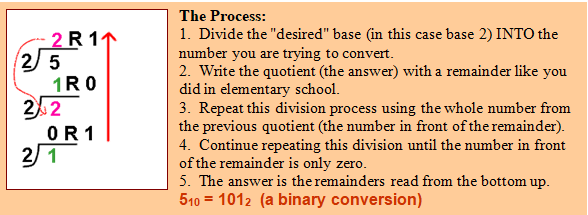Example 2:  Convert 14010 to base 8.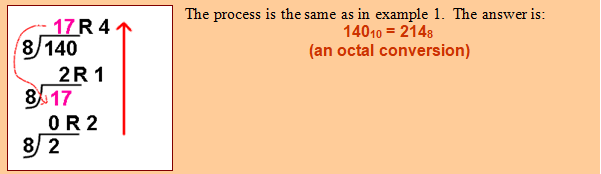Example 3:  Convert 11010 to base 16.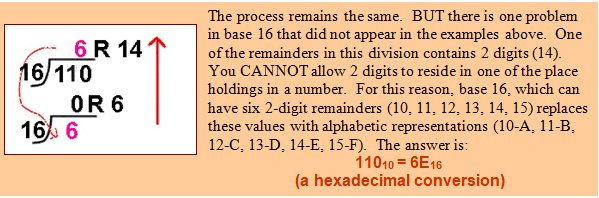Card Game

Choose a number from 1 to 60. You will find it on one or more of the cards below. Show them. Make sure you don’t miss any.

Then I can reveal your number.

While it looks impressive, it relies on simple Mathematics.

Ask someone to think of a number. They should find all the cards with that number on, and give them to you. All you need do is add up the top left hand number on each of these cards. This will be the number they chose.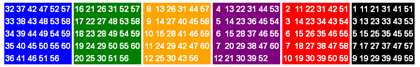Can you explain this magic trick ?

## Related files

 1 NUMERAL SYSTEMS_students 528 KB 2 NUMERAL SYSTEMS exercises 428 KB 3 NUMERAL SYSTEMS change of base 363 KB Numeral systems 385 KB numeral systems _ Card Game 363 KB
If you have tried out a lesson, please fill the next form to help us to improve it: